Smartick is an online platform for children to master math in only 15 minutes a day

Oct06

In previous posts, we’ve looked at what prime numbers are, and how to factorize them. In this post, we’re going to teach you a trick to help you work out whether a number is prime or not, without having to work out its divisors. This method is much more fun, and will also help you find its divisors if there are any…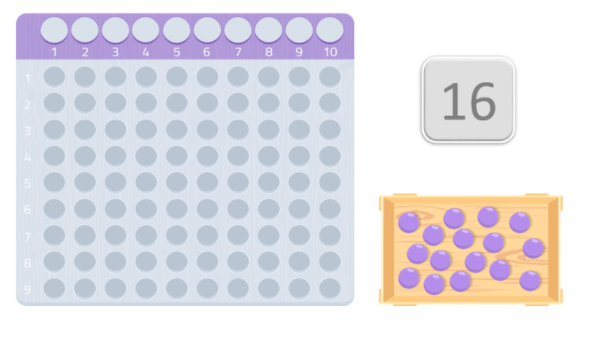We’ll choose a random number… for example 16. To work out whether or not it’s a prime number, we’re going to use a table – similar to the Montessori multiplication table. We’re going to add the same number of balls to the table as the number we have chosen, in this case, 16.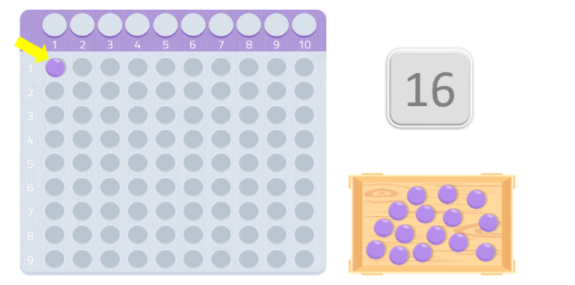Once we have everything ready, we need to put the balls in the table, starting from the first space, and trying to make the shape of a rectangle. The numbers at the corners of the rectangle will be the divisors of the number. If we get a rectangle that shows the number we’re using and one, it will be a prime number.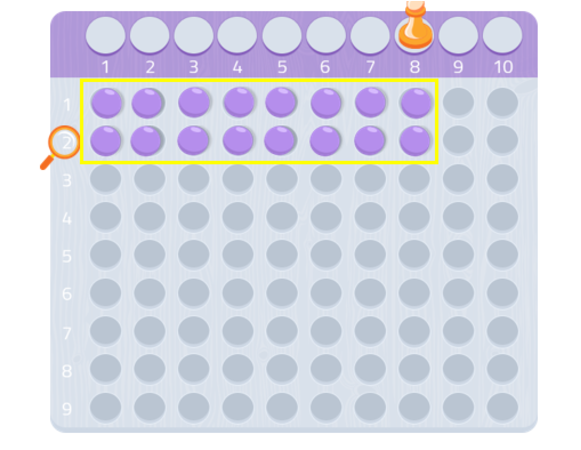For example, in this case, we’ve put 8 balls in the first row, and 8 more in the second. As you can see, we’ve made a rectangle, and we can see that both 8 and 2 are divisors of the number 16. So the number 16 is not a prime number. As we already know, prime numbers are numbers that are only divisible by 1 and themselves.

Now let’s try another number, 7, for example: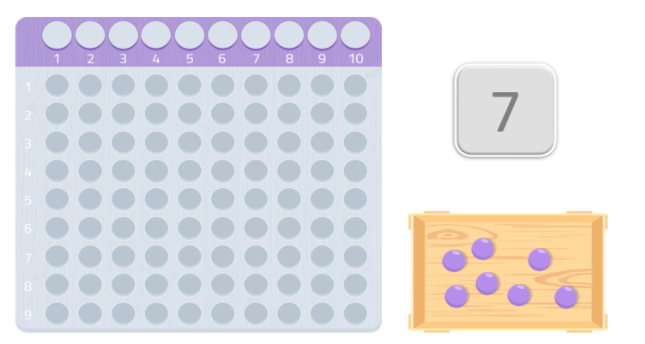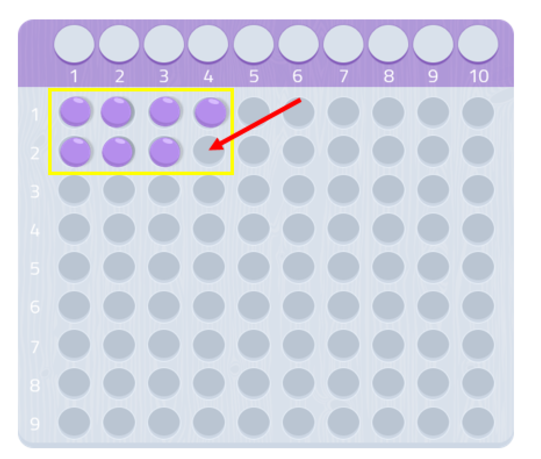As we can see, we can’t make a complete rectangle… There’s a ball missing! Since we can’t make a rectangle, we can say that the number 7 doesn’t have any divisors, apart from itself and 1, as we can see in the following image. Therefore, 7 is a prime number!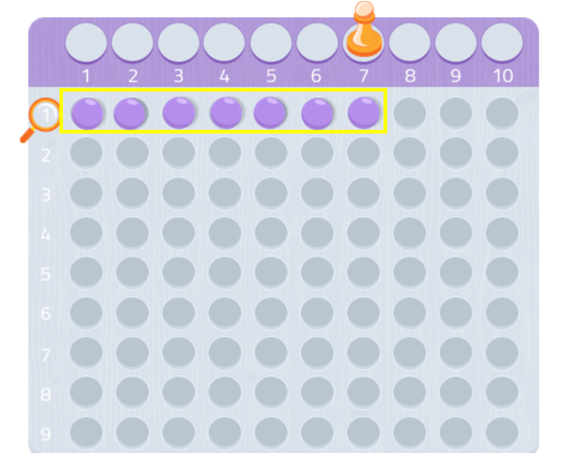What will happen if we try it with the number 9? Let’s find out…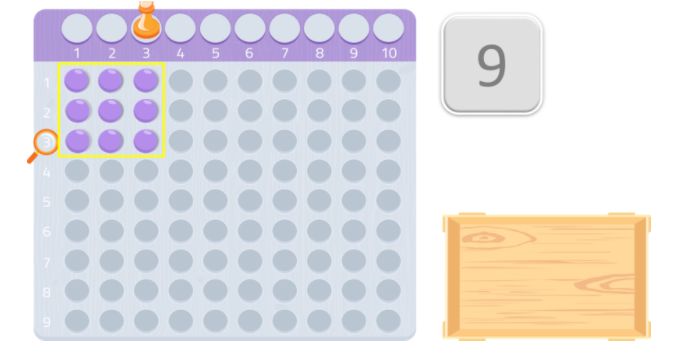As we can see, the number 9 makes a square. In mathematics, the square is considered a type of rectangle that, in addition to having four right angles like all rectangles, has four equal sides. Therefore, 9 is not a prime number (we can make a rectangle with it), and its divisors are 1, 3, and 9.

Try it with any number!! It really works!!